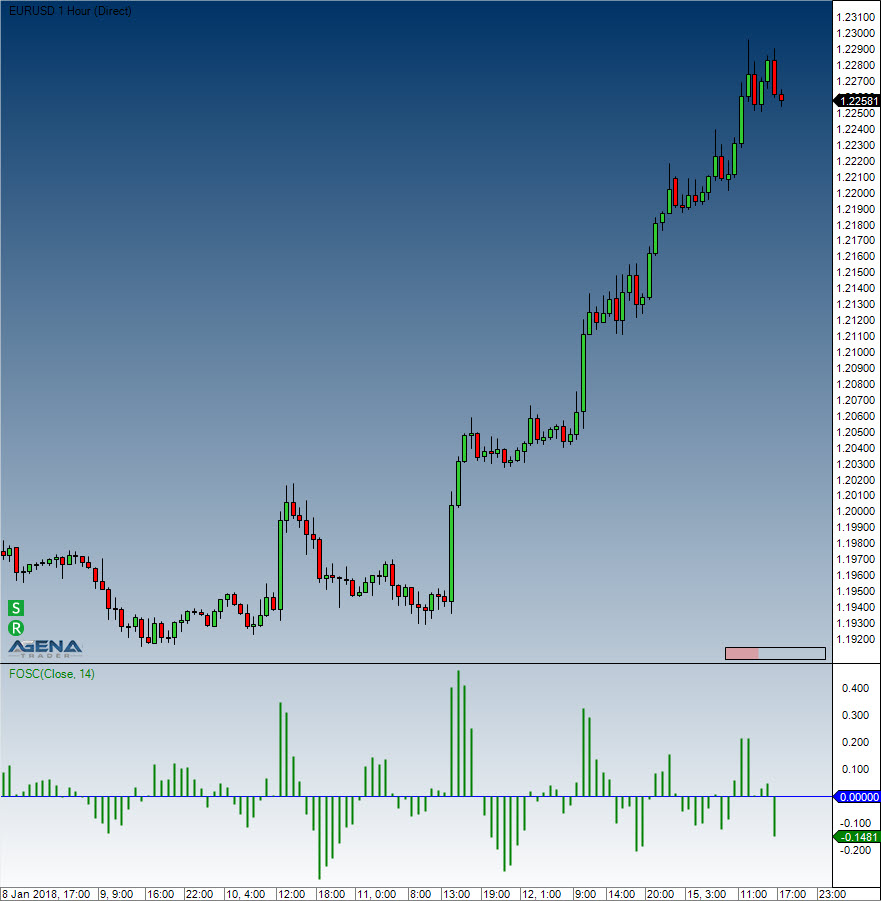Comment on page

# Forecast Oscillator (FOSC)

#### Description

The FOSC makes a comparison of the current price against the value returned by the Time Series Forecast study. It is calculated as a percentage ratio of the difference between the close price and the Time Series Forecast value for the previous bar.
When this oscillator displays positive values, it hints that the Time Series Forecast has underestimated the price, whereas negative values suggest that the TSF has overestimated the price. Usually, an SMA also accompanies the Forecast Oscillator line in the search for oscillator reversals.

#### Parameters

inSeries Input data series for the indicator
period Number of bars included in the calculations

#### Return value

double
When using this method with an index (e.g. FOSC(14)[int barsAgo] ), the value of the indicator will be issued for the referenced bar.

#### Usage

FOSC(int period)
FOSC(IDataSeries inSeries, int period)
FOSC(int period)[int barsAgo]
FOSC(IDataSeries inSeries, int period)[int barsAgo]

#### VisualizationForecast Oscillator (FOSC)

#### Examples

//Output for the Forecast Oscillator
Print("The current value for the Forecast Oscillator is: " + FOSC(14));
//Determining the future market direction
if (FOSC(14) > 0)
Print("The FOSC is bigger than zero, which indicates rising price movements.");